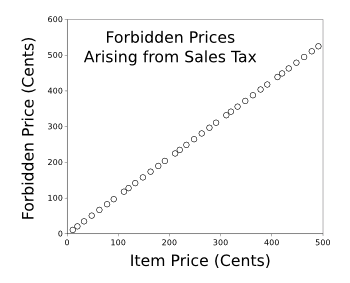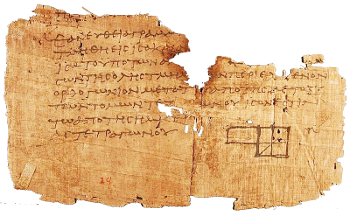### Avoided Numbers

May 12, 2016

New York State initiated its sales tax on April 14, 1965, while I was there attending high school. At its initiation, this tax was just 2%, but it quickly ramped up over the years, with counties adding their own sales taxes. Fortunately, many items are excluded from sales tax, including funeral expenses. In a small sense, you can take it with you.

When this sales tax was initiated, I realized that it would be impossible to buy some items at certain prices. As an example, consider the sales tax for New Jersey, presently at 7%. There is no sales tax on an item costing ten cents, but there's a one cent sales tax on an item costing eleven cents. That means that you can't pay eleven cents for an item. You can only buy that item for twelve cents. Eleven is a forbidden number.

Because of this sales tax, it's impossible to buy items for 11, 21, 35, 51, 67, 83, or 97 cents. As the following graph shows, there are seven forbidden prices in each dollar interval.Forbidden prices for goods in New Jersey as a consequence of its 7% sales tax.

(Graphed using Gnumeric.)

While seven is considered to be a lucky number by some people, it was a number to be avoided in certain cultures under certain circumstances. When doubly cursed by seven, as being the seventh son of a seventh son, you might be a vampire. Fortunately, science has dispelled such numerology, although some physicists think that it still persists in string theory.

Consider a person who's so afraid of the number, seven, that he refuses to leave his house on the seventh day of each month. Moreover, he chooses to remain inside on the 17th and 27th of each month. A little strange, but he could still function in the modern world by making up lost work days on weekends.

Then comes the month of July, the seventh month. He would need to stock a lot of food, and take that month as his work vacation. He's still able to function in our modern world. His big problem arises next year, 2017. While we leave our subject's researching use of the Hebrew calendar and other calendars as a possible remedy, it's interesting to consider how many numbers we would need to reject from the natural numbers if we wished to avoid a particular digit.

Often it's easier to calculate something in the converse, so we'll calculate the probability that a number does not contain a particular digit. For numbers from 0-9, this would be 9/10. For a two digit number, we need to multiply this probability to get (81/100), which means we should expect 19 numbers in that range to contain a seven, which is verified by inspection. As the following graph shows, it becomes more difficult to find a number devoid of the digit, seven, as we go to larger numbers.Probability that a number will not contain a particular digit (for example, seven) as a function of the number of its digits.

(Graphed using Gnumeric.)

This result is simply expressed as p(n) = (9/10)n, where n is the number of digits of the number, and it can be expressed in an arbitrary base b as p(n) = (b-1/b)n. Obviously, the curve for hexadecimal numbers (base-16) will descend less quickly, and octal numbers (base-8) will have a steeper curve, as shown below.Avoided digit probability for base-16, base-10, and base-8.

(Graphed using Gnumeric.)

To find how many numbers fulfill our avoided-digit criterion up to a number N, we need to integrate under these curves. Lazy mathematician that I am, I resort to the result given in a recent arXiv paper by James Maynard.

The numbers fulfilling the avoided-digit criterion up to N are of the order, O(N(1-c)), where c = log(b/(b-1))/log(b). For decimal numbers, c ≈ 0.045757. Although the probability is low, up to 100 decimal digits we still obtain 2.65 x 1095 numbers meeting our avoided digit criterion.

In his arXiv paper, Maynard addressed the question of whether there are an infinity of prime numbers in the set of such avoided-digit numbers. The infinity of primes among all the natural numbers was established by Euclid in his Elements, Book IX, Proposition 20, a proof that's easy enough for a high school student to understand.